# Deltahedron

Not to be confused with Deltohedron.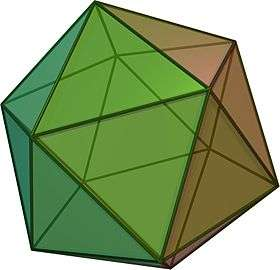The largest strictly-convex deltahedron is the regular icosahedron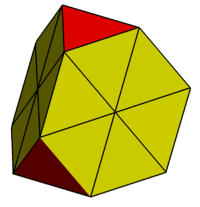This is a truncated tetrahedron with hexagons subdivided into triangles. This figure is not a strictly-convex deltahedron since coplanar faces are not allowed within the definition.

A deltahedron (plural deltahedra) is a polyhedron whose faces are all equilateral triangles. The name is taken from the Greek majuscule delta (), which has the shape of an equilateral triangle. There are infinitely many deltahedra, but of these only eight are convex, having 4, 6, 8, 10, 12, 14, 16 and 20 faces.(Freudenthal 1947) The number of faces, edges, and vertices is listed below for each of the eight convex deltahedra.

## The eight convex deltahedra

There are only eight strictly-convex deltahedra: three are regular polyhedra, and five are Johnson solids.

Regular deltahedra
ImageName Faces Edges Vertices Vertex configurations Symmetry group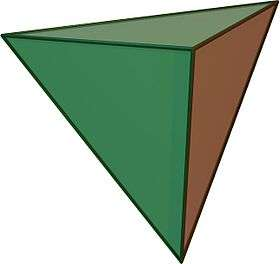tetrahedron 4 6 4 4 × 33 Td, [3,3]octahedron 8 12 6 6 × 34 Oh, [4,3]icosahedron 20 30 12 12 × 35 Ih, [5,3]
Johnson deltahedra
ImageName Faces Edges Vertices Vertex configurations Symmetry group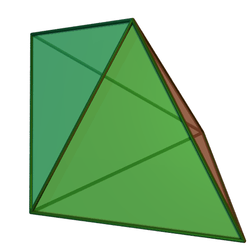triangular bipyramid 6 9 5 2 × 33
3 × 34
D3h, [3,2]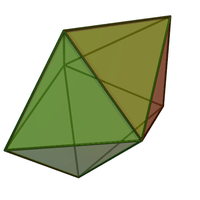pentagonal bipyramid 10 15 7 5 × 34
2 × 35
D5h, [5,2]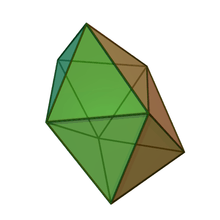snub disphenoid 12 18 8 4 × 34
4 × 35
D2d, [2,2]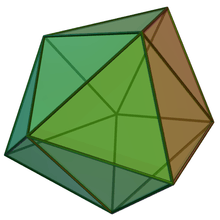triaugmented triangular prism 14 21 9 3 × 34
6 × 35
D3h, [3,2]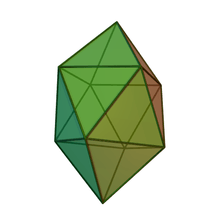gyroelongated square bipyramid 16 24 10 2 × 34
8 × 35
D4d, [4,2]

In the 6-faced deltahedron, some vertices have degree 3 and some degree 4. In the 10-, 12-, 14-, and 16-faced deltahedra, some vertices have degree 4 and some degree 5. These five irregular deltahedra belong to the class of Johnson solids: convex polyhedra with regular polygons for faces.

Deltahedra retain their shape, even if the edges are free to rotate around their vertices so that the angles between edges are fluid. Not all polyhedra have this property: for example, if you relax some of the angles of a cube, the cube can be deformed into a non-right square prism.

There is no 18-faced convex deltahedron. However, the edge-contracted icosahedron gives an example of an octadecahedron that can either be made convex with 18 irregular triangular faces, or made with equilateral triangles that include two coplanar sets of three triangles.

## Non-strictly-convex cases

There are infinitely many cases with coplanar triangles, allowing for sections of the infinite triangular tilings. The coplanar triangular faces can be merged into rhombic, trapezoidal, hexagonal, or other equilateral polygon faces. If the sets of coplanar triangles are considered a single face (called a triamond), a smaller set of faces, edges, and vertices can be counted. Triamond faces used must be convex, including: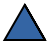,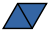,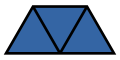,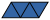,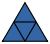,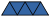,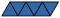and, ...

Some smaller examples include:

Coplanar deltahedra
Image Name Faces Edges Vertices Vertex configurations Symmetry group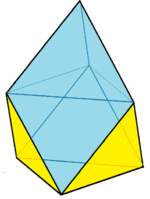Augmented octahedron
Augmentation
1 tet + 1 oct
1015 7 1 × 33
3 × 34
3 × 35
0 × 36
C3v, 
4312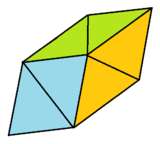Trigonal trapezohedron
Augmentation
2 tets + 1 oct
1218 8 2 × 33
0 × 34
6 × 35
0 × 36
C3v, 
612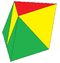Augmentation
2 tets + 1 oct
12188 2 × 33
1 × 34
4 × 35
1 × 36
C2v, 
222117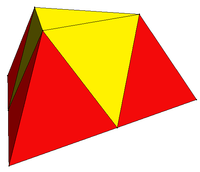Triangular frustum
Augmentation
3 tets + 1 oct
14219 3 × 33
0 × 34
3 × 35
3 × 36
C3v, 
13196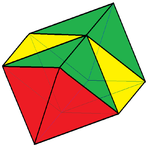Elongated octahedron
Augmentation
2 tets + 2 octs
162410 0 × 33
4 × 34
4 × 35
2 × 36
D2h, [2,2]
44126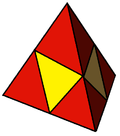Tetrahedron
Augmentation
4 tets + 1 oct
162410 4 × 33
0 × 34
0 × 35
6 × 36
Td, [3,3]
464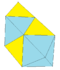Augmentation
3 tets + 2 octs
182711 1 × 33
2 × 34
5 × 35
3 × 36
D2h, [2,2]
2122149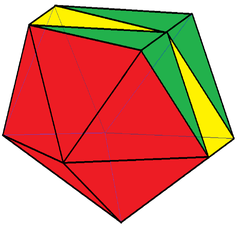Edge-contracted icosahedron 182711 0 × 33
2 × 34
8 × 35
1 × 36
C2v, 
1222210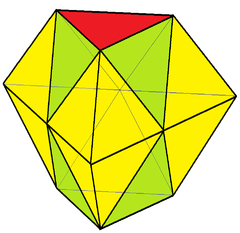Triangular bifrustum
Augmentation
6 tets + 2 octs
203012 0 × 33
3 × 34
6 × 35
3 × 36
D3h, [3,2]
26159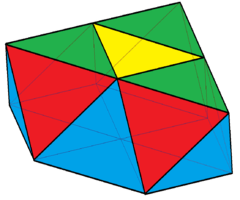triangular cupola
Augmentation
4 tets + 3 octs
223313 0 × 33
3 × 34
6 × 35
4 × 36
C3v, 
3311159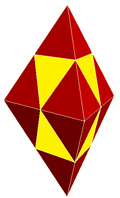Triangular bipyramid
Augmentation
8 tets + 2 octs
243614 2 × 33
3 × 34
0 × 35
9 × 36
D3h, 
695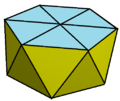Hexagonal antiprism 243614 0 × 33
0 × 34
12 × 35
2 × 36
D6d, [12,2+]
1222412Truncated tetrahedron
Augmentation
6 tets + 4 octs
284216 0 × 33
0 × 34
12 × 35
4 × 36
Td, [3,3]
441812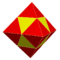Tetrakis cuboctahedron
Octahedron
Augmentation
8 tets + 6 octs
322418 0 × 33
12 × 34
0 × 35
6 × 36
Oh, [4,3]
8126

## Non-convex forms

There are an infinite number of nonconvex forms.

Some examples of face-intersecting deltahedra:

Other nonconvex deltahedra can be generated by adding equilateral pyramids to the faces of all 5 regular polyhedra:

 triakis tetrahedron tetrakis hexahedron triakis octahedron(stella octangula) pentakis dodecahedron triakis icosahedron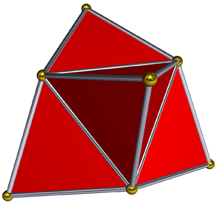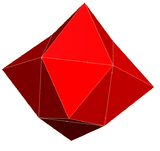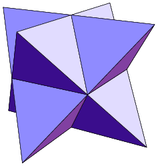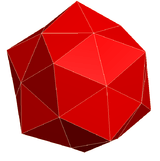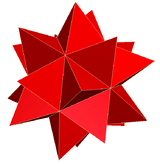Other augmentations of the tetrahedron include:

8 triangles 10 triangles 12 triangles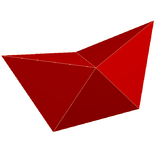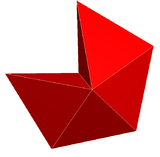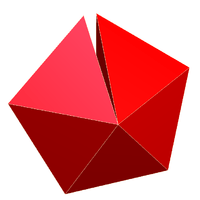Also by adding inverted pyramids to faces:

60 triangles 48 triangles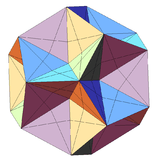Excavated dodecahedron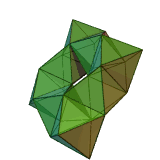A toroidal deltahedron

## References

1. Trigg, Charles W. (1978), "An Infinite Class of Deltahedra", Mathematics Magazine, 51 (1): 55–57, JSTOR 2689647.
2. The Convex Deltahedra And the Allowance of Coplanar Faces
3. http://www.interocitors.com/polyhedra/Triamonds/
• Freudenthal, H; van der Waerden, B. L. (1947), "Over een bewering van Euclides ("On an Assertion of Euclid")", Simon Stevin (in Dutch), 25: 115–128 (They showed that there are just 8 convex deltahedra. )
• O. Rausenberger Konvexe pseudoreguläre Polyeder. Zeitschrift für mathematischen und naturwissenschaftlichen Unterricht 46, 135-142, 1915.
• H. Martyn Cundy Deltahedra. Math. Gaz. 36, 263-266, Dec 1952.
• H. Martyn Cundy and A. Rollett Deltahedra. §3.11 in Mathematical Models, 3rd ed. Stradbroke, England: Tarquin Pub., pp. 142–144, 1989.
• Charles W. Trigg An Infinite Class of Deltahedra, Mathematics Magazine, Vol. 51, No. 1 (Jan., 1978), pp. 55–57
• M. Gardner Fractal Music, Hypercards, and More: Mathematical Recreations, Scientific American Magazine. New York: W. H. Freeman, pp. 40, 53, and 58-60, 1992.
• Anthony Pugh (1976). Polyhedra: A visual approach. California: University of California Press Berkeley. ISBN 0-520-03056-7. pp. 35–36